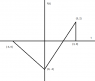# Sketch graph as function of another

• geft

#### geft

Sketch g(t) = f(-3t + 9).

I know how to work this out with trial and error:

t = 0 => g(t) = f(9) = 0
t = 1 => g(t) = f(6) = 0
t = 2 => g(t) = f(3) = 2
t = 3 => g(t) = f(0) = -3
t = 4 => g(t) = f(-3) = 0
etc...

How do I do this in a faster and more reliable way, taking into account all the coordinates?

#### Attachments

•Untitled.png
2.3 KB · Views: 404